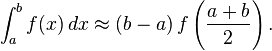# How to estimate roll position via gyroscope data?

usb232
im trying to implement a tilt sensing program via accelerometer and gyroscope. part of the input that i will plan on using is gyroscope data. since gyroscopes measure angle/sec, integrating this will give me angle right? i then have three questions:

1. do i multiply the gyroscope's (1/sampling rate) to the deg/sec to get degrees?
2. if i am to do the first one, for lack of a better term am i going to "discretize" the value? that is, any angle measurement i take is multiplied to a constant = (1/sampling rate) OR
3. do i do something like this 1/ (sampling rate*n) where n is the sample number?

thank you!

serbring
im trying to implement a tilt sensing program via accelerometer and gyroscope. part of the input that i will plan on using is gyroscope data. since gyroscopes measure angle/sec, integrating this will give me angle right? i then have three questions:

It's right

1. do i multiply the gyroscope's (1/sampling rate) to the deg/sec to get degrees?
2. if i am to do the first one, for lack of a better term am i going to "discretize" the value? that is, any angle measurement i take is multiplied to a constant = (1/sampling rate) OR
3. do i do something like this 1/ (sampling rate*n) where n is the sample number?

thank you!

take a look to this
http://en.wikipedia.org/wiki/Numerical_integration

Remember that numerical integration imply a numerical drift in steady signals

Hope this helps

usb232
i would like to begin by saying thanks!
ive read through the article but my understanding of it is not that good. im sorry.

it says in this part that to use
rectangle rule
for integration i am to use this formula:however it requires that i evaluate at points a and b. the only data i have is (b-a) which is delta, or the gyroscope's sampling rate. does this mean that i wont be able to estimate the angle?

serbring
i would like to begin by saying thanks!
ive read through the article but my understanding of it is not that good. im sorry.

it says in this part that to use for integration i am to use this formula:however it requires that i evaluate at points a and b. the only data i have is (b-a) which is delta, or the gyroscope's sampling rate. does this mean that i wont be able to estimate the angle?

Maybe I'm misunderstanding what you have written, but f(a) and f(b) are the sampled values of angle rate, so you have all data you need

usb232
sorry about that. i should have given a clear example.

1. suppose at time ta i get a converted gyro reading of 20 deg/sec. i then multiply this to (1/gyro_sampling rate) = 2seconds to get 40 degs ----> this is f(a)?
2. at time tb i get a converted reading of 30 deg/sec. i again multiply this to 2 seconds to get 60 degrees ----> f(b)?
3. what would be my (b-a)?
4. how do i calculate f ( (a+b) / 2)? i dont have the function f T_T

serbring
sorry about that. i should have given a clear example.

1. suppose at time ta i get a converted gyro reading of 20 deg/sec. i then multiply this to (1/gyro_sampling rate) = 2seconds to get 40 degs ----> this is f(a)?
2. at time tb i get a converted reading of 30 deg/sec. i again multiply this to 2 seconds to get 60 degrees ----> f(b)?
3. what would be my (b-a)?
4. how do i calculate f ( (a+b) / 2)? i dont have the function f T_T

if at t=ta you read 20 deg/s and at t=tb you read 30 deg/s, a=ta, f(a)=20, b=tb, f(b)=30.

Hope this helps

usb232
am i right in assuming that since (1/sampling rate) = delta then
ta = a = n * delta and
tb = b = (n+1) *delta
then b-a = delta too?

and f( (a+b) / 2) = (20 + 30) / 2 = 25?

serbring
am i right in assuming that since (1/sampling rate) = delta then
ta = a = n * delta and
tb = b = (n+1) *delta
then b-a = delta too?

and f( (a+b) / 2) = (20 + 30) / 2 = 25?

that's true